Light refraction and reflection
Date of measure: 11.04.2019

 Group A Jakub, Lucka, Wendy, Simona, Pepa Group B Jan, Lukáš, Vojta, Kača Group C Market, Market, Kiki, Kiki, Eli a Amča Group D Rozka, Marťa, Vendy, Ondra, Vojta B. Group E Jirka, Petr, Ríša, Marek, Honza Translators Vendy M., Markéta L., Laura

### Tools

Virtual laboratory

1. ### Light refraction to the perpendicular line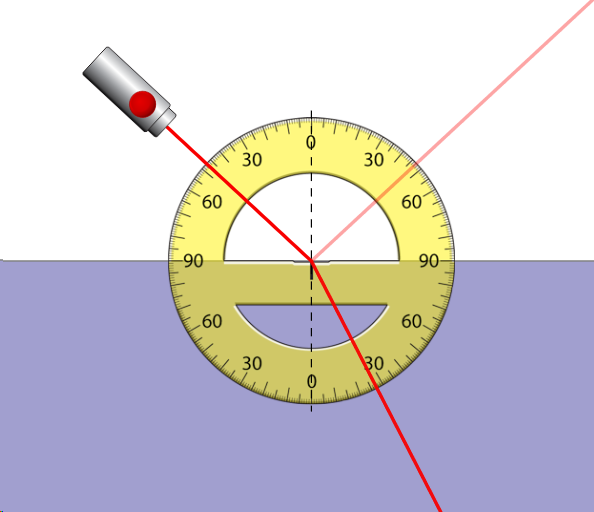Group A
first substance:air n
1=1
second substance: n
2 = 1,45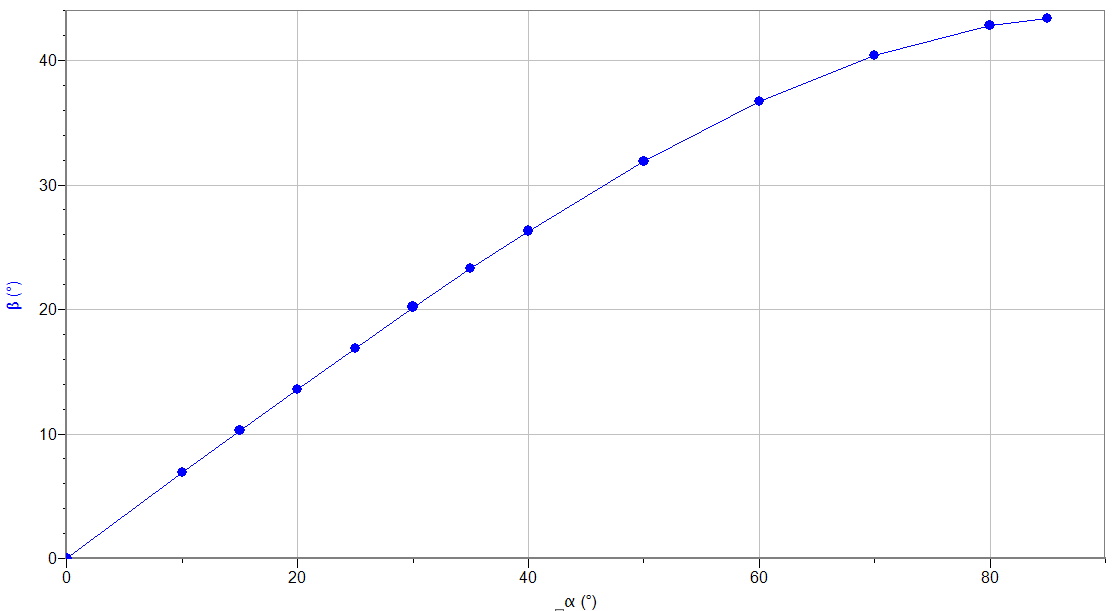Group B
first substance: n
1= 1
second substance: n
2 = 1,4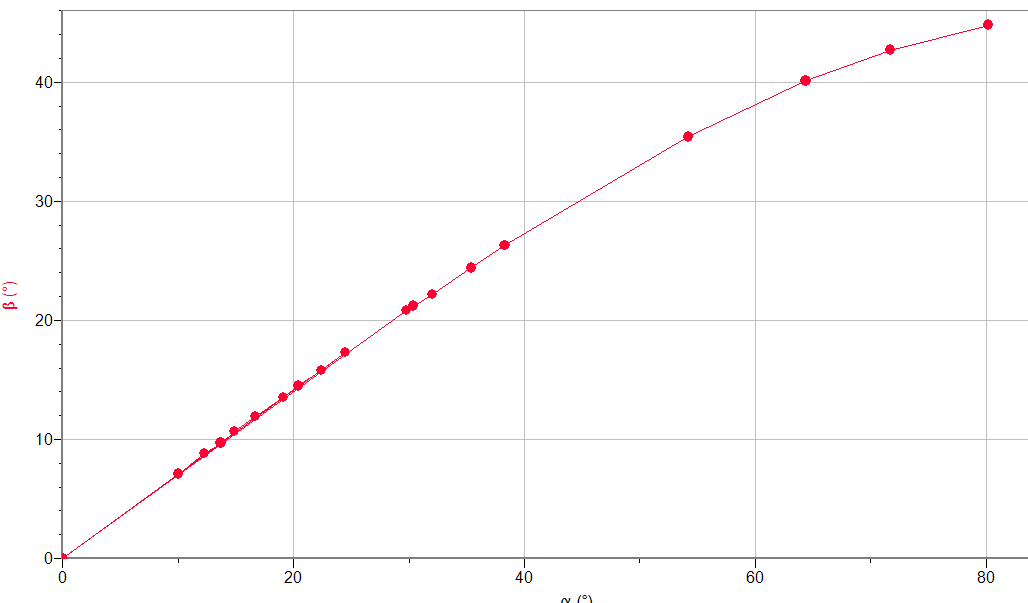Group C
first substance:air n
1=1
second substance: n
2 = 1,5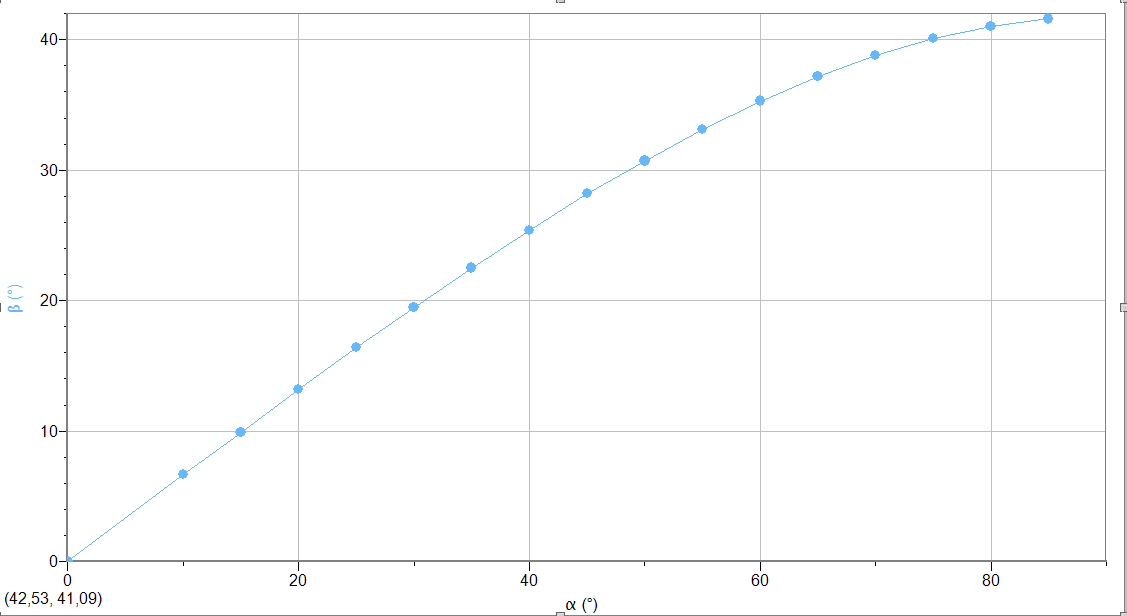Group D

first substance:air n1=1
second substance: n
2 = 1,6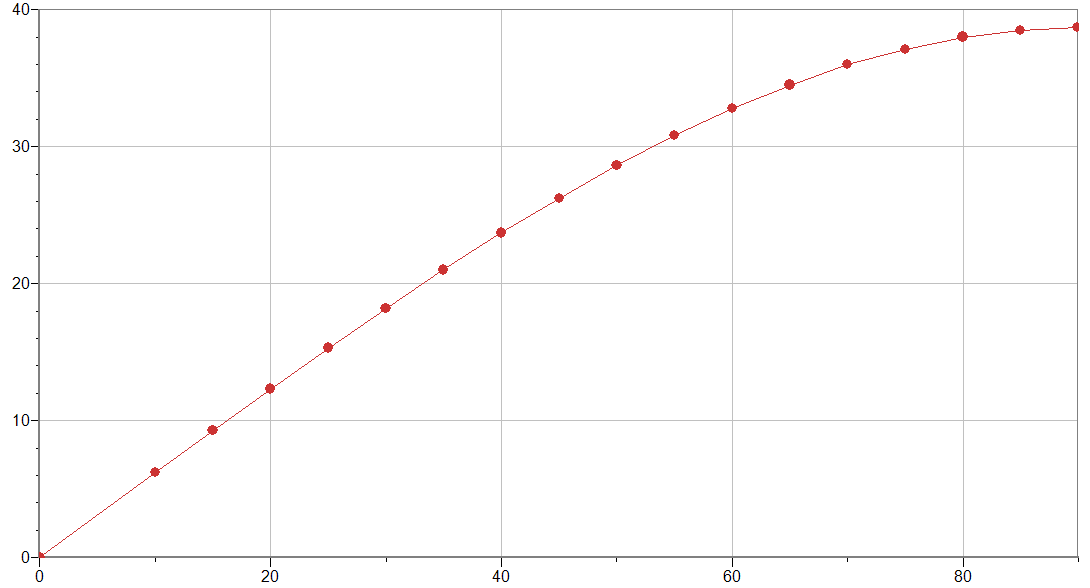Group E

first substance:air n1=1
second substance: n
2 = 1,333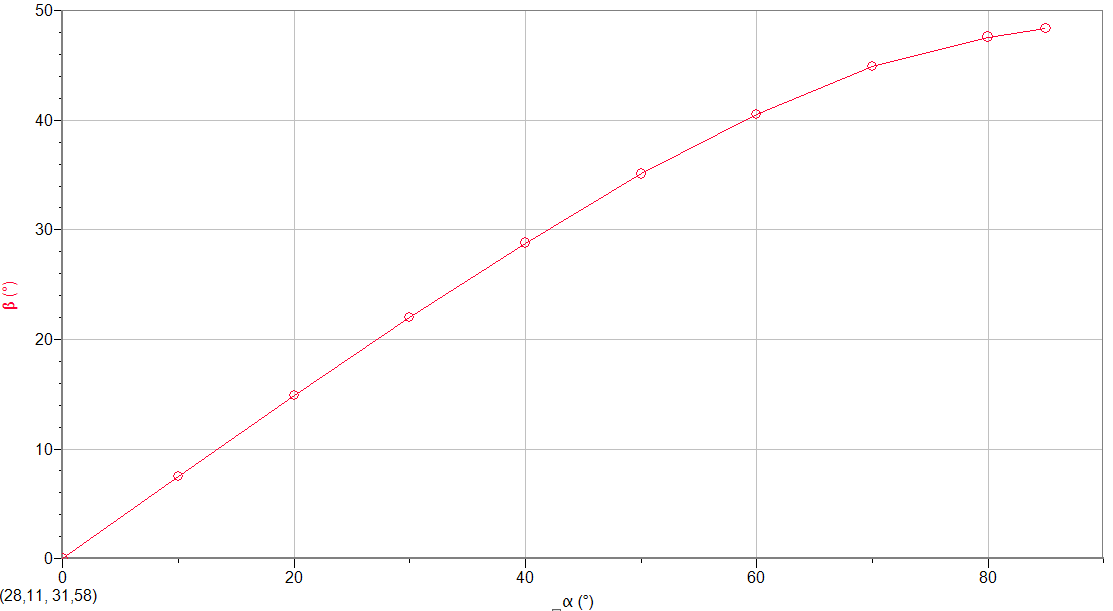1. ### Light reflection in the interface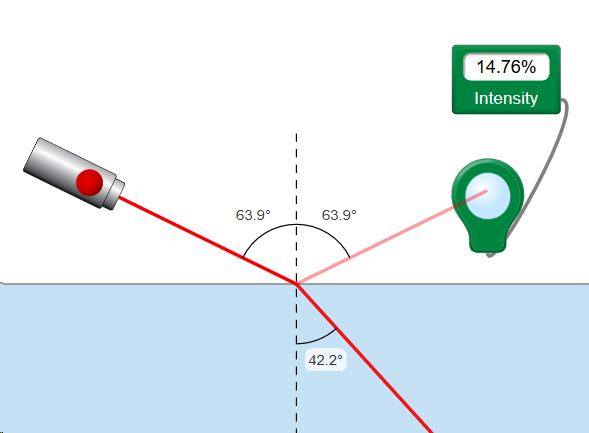Group A
first substance:air n
1=1
second substance: n
2 = 1,45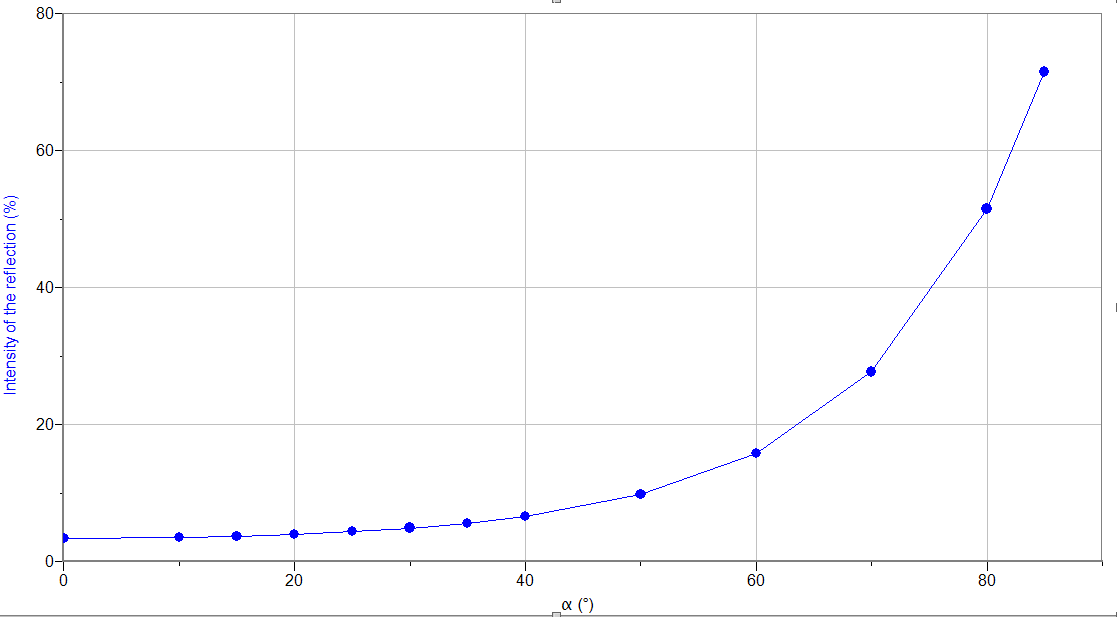Group  B
first substance:air n
1=1
second substance: n
2 = 1,3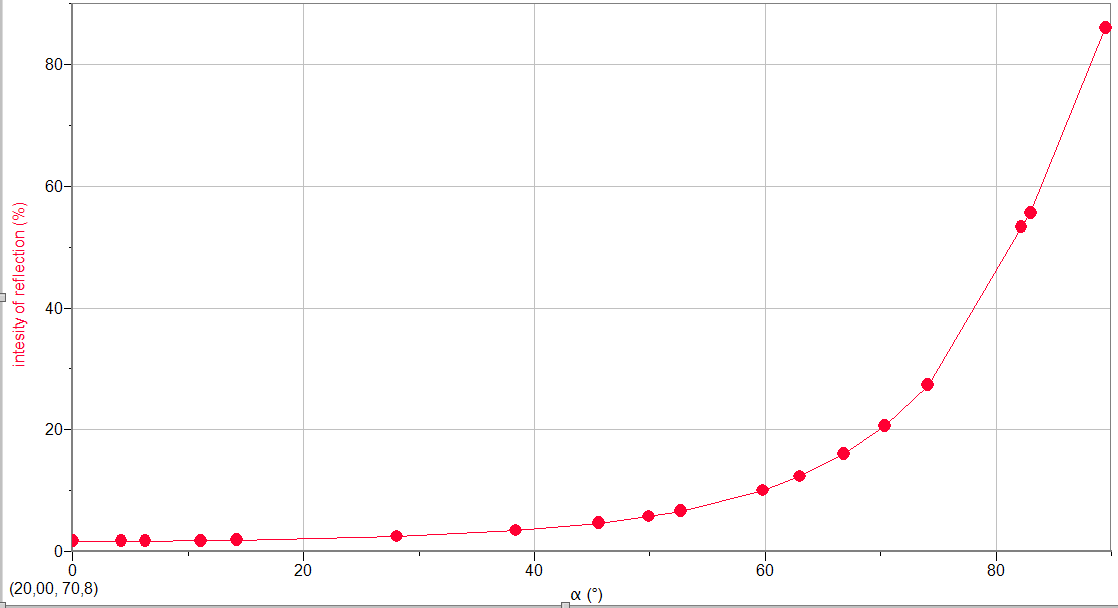Group C
first substance:air n
1=1
second substance: n
2 = 1,33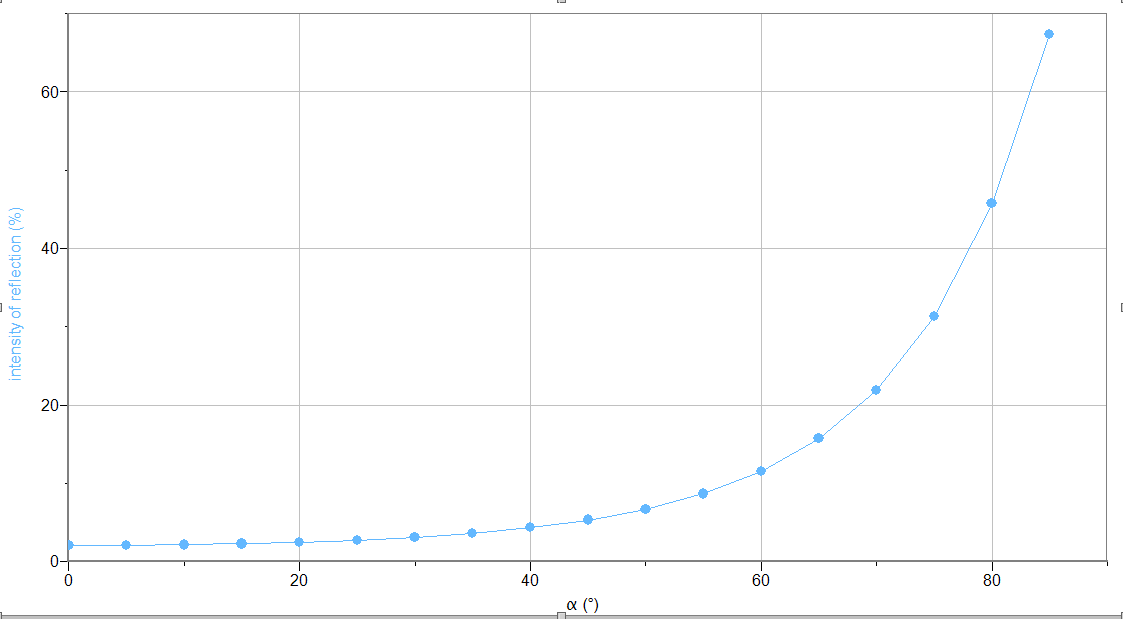Group D
first substance:air n
1= 1,00
second substance: n
2 = 1,60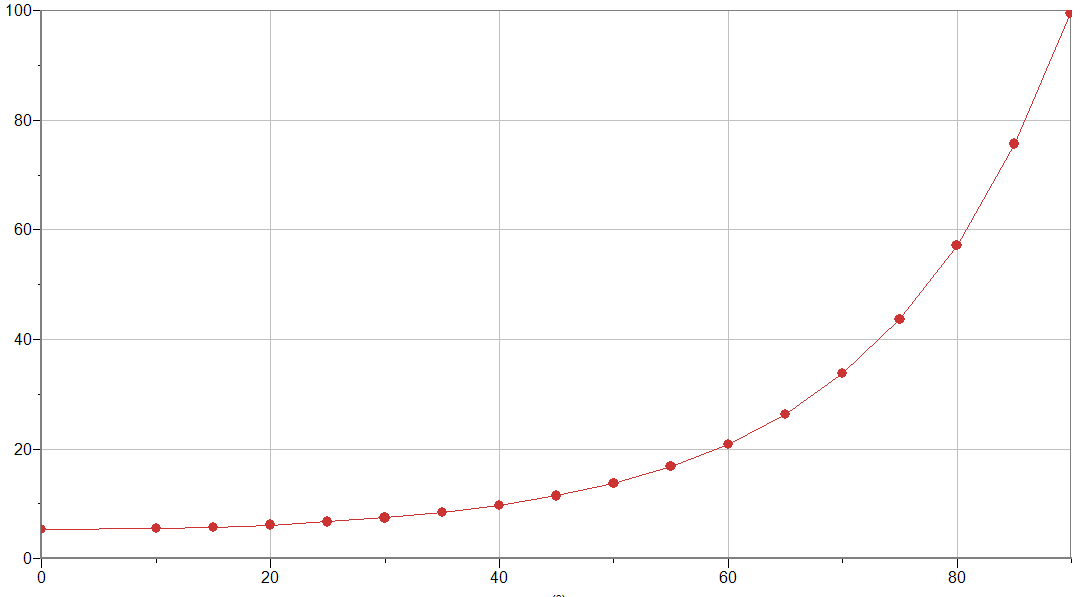Group E
first substance:air n
1=1
second substance: n
2 = 1,4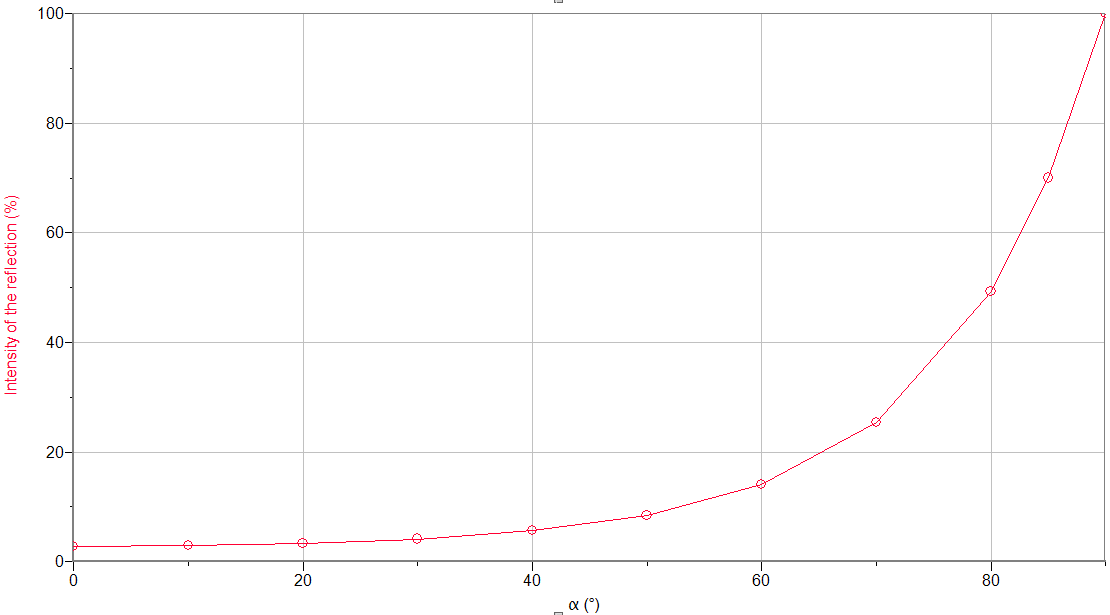….

1. ### Total reflection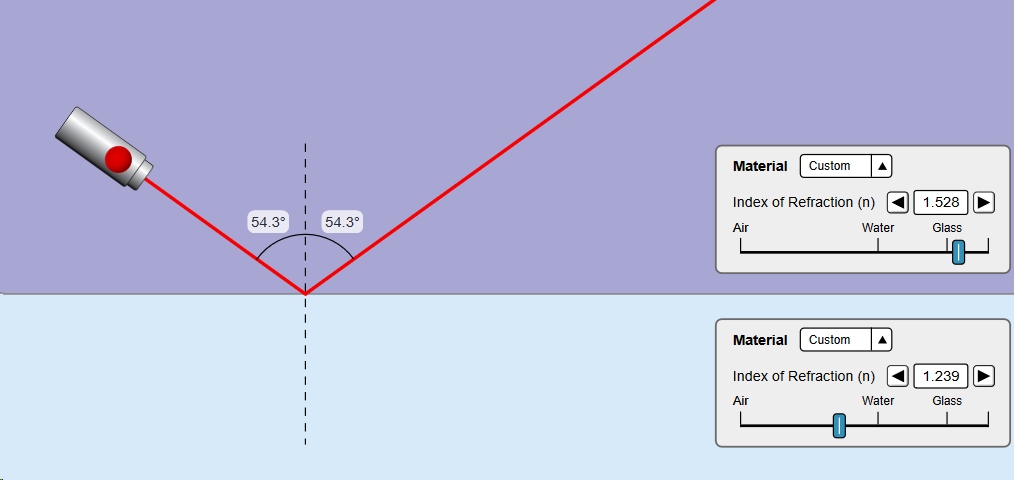Group n1 n2 terminal angle A 1,50 1,30 60,4 B 1,41 1,00 45,5 C 1,50 1,33 62,8 D 1,60 1,00 38,7 E 1,33 1,00 48,6

The End